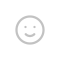## RSA算法的实现

``````#RSA算法接收方程序，by小草 https://xcao.top/?p=222
import random

def gcd(a, b):
while b != 0:
a, b = b, a % b
return a

def coprime(a, b):
return gcd(a, b) == 1

# 两个函数作用均为判断两数是否互质

p = 0
q = 0
while p == q:
p = random.choice([2, 3, 5, 7, 11, 13, 17, 19])
q = random.choice([2, 3, 5, 7, 11, 13, 17, 19])
n = p * q
n1 = (p - 1) * (q - 1) #φ(n)不方便打，以n1代替

# 随机取p和q（此处只取20以内的质数方便计算，并计算出n以及φ(n)
while True:
e = random.randint(1, n1)
if coprime(e, n1):
break
for i in range(1, 10000):
d = (i * n1 + 1) / e
if e*d > n1:
if d % 1 == 0:
d = int(d)
break
print('公钥是',e)
print('两质数积为',n)
# 计算出公钥以及私钥

c = int(input('请输入密文\n'))
m = pow(c,d)
m = m % n
print('明文是',m)
``````
``````#RSA算法发送方程序，by小草 https://xcao.top/?p=222
e = int(input('请输入公钥\n'))
n=int(input('请输入两质数积\n'))
m = int(input('请输入明文\n'))
c = pow(m,e)
c = c % n
print('密文是',c)
``````

## 评论

1.Windows Chrome 94.0.4606.81
2年前
2021-11-28 17:21:30

写得好，所以我选择ECDSA•博主
Windows Chrome 96.0.4664.45
2年前
2021-11-28 17:25:18

看看能不能给ECDSA也写一篇文章## 发送评论编辑评论

|´・ω・)ノ
ヾ(≧∇≦*)ゝ
(☆ω☆)
（╯‵□′）╯︵┴─┴
￣﹃￣
(/ω＼)
∠( ᐛ 」∠)＿
(๑•̀ㅁ•́ฅ)
→_→
୧(๑•̀⌄•́๑)૭
٩(ˊᗜˋ*)و
(ノ°ο°)ノ
(´இ皿இ｀)
⌇●﹏●⌇
(ฅ´ω`ฅ)
(╯°A°)╯︵○○○
φ(￣∇￣o)
ヾ(´･ ･｀｡)ノ"
( ง ᵒ̌皿ᵒ̌)ง⁼³₌₃
(ó﹏ò｡)
Σ(っ °Д °;)っ
( ,,´･ω･)ﾉ"(´っω･｀｡)
╮(╯▽╰)╭
o(*////▽////*)q
＞﹏＜
( ๑´•ω•) "(ㆆᴗㆆ)

Emoji